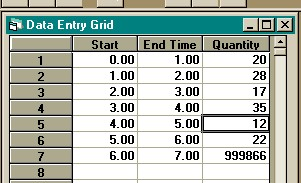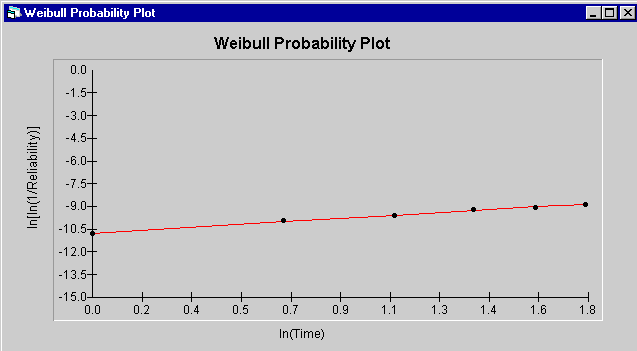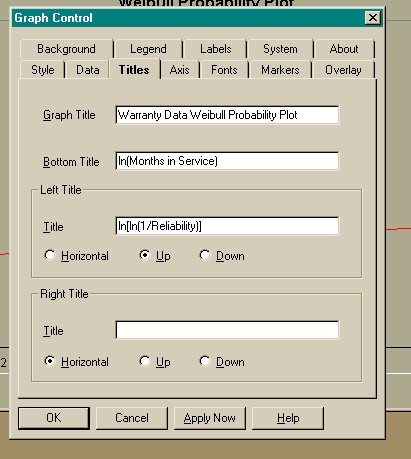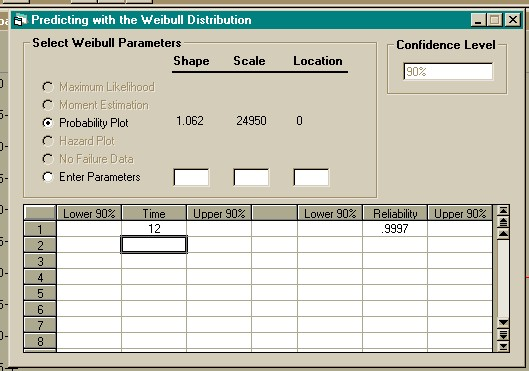Example 2
Home

Life Data Analysis

One million units were introduced into the field 6 months ago.  The number of failures per month are shown below.

 Month Ending Number of Failures 1 20 2 28 3 17 4 35 5 12 6 22

What is the expected reliability of the population after 1 year in service?

### Solution

1. This data is in interval format.  Select "New" then "Grouped Data" from the "File" menu.  And enter the data as shown below.2. Row 7 of the grouped data input spreadsheet shown above does NOT indicate that 999,866 units failed in the period from 6 months to 7 months.  The last row of the spreadsheet always contains the number of units that have not failed.

3. Select "Weibull" then "Probability Plot" from the "Parameter Estimation" menu to obtain a probability plot estimation.  Then click the "Plot" button to obtain the plot.  This is shown in the figure below.  When using grouped data, the only option for estimation is probability plotting.  Because of the large sample size with grouped data, probability plotting is as accurate as maximum likelihood estimation.  The large sample size also eliminates the need for confidence intervals.  The estimated values are nearly the same as lower or upper confidence levels.4. If the default graph is not in the format you want, right click on the graph, and the dialog box shown below will allow you to customize the colors, title, background, colors, etc.5. The points on the probability plot follow the straight line, so the Weibull distribution is accepted as a model.  Select "Weibull" from the "Predictions" menu.  Click the spreadsheet in the first row under the "Time" column and enter 12.  After entering 12 press the down cursor or press the "Enter" key.  The expected reliability at 1 year in service (12 months) is 0.9997.  This is shown below.